# The Kármán Line

The Kármán Line, at 100 kilometres above sea level, is commonly taken to be the boundary between Earth’s atmosphere and space. But why?

An aircraft generates lift by moving through air.$L=\frac{1}{2} AC_L \rho v^2$

where$L$ is the lift generated,$A$ is the wing area,$C_L$ is the lift coefficient,$\rho$ is the density of air, and$v$ is speed through the air.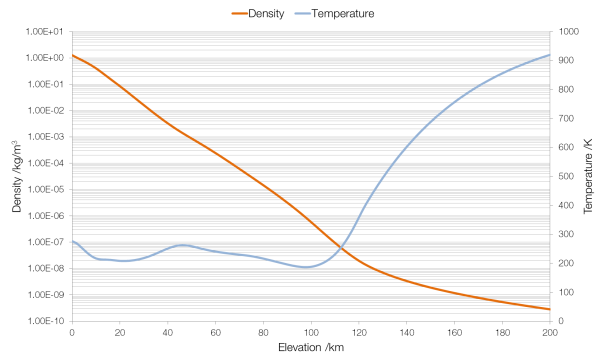Data from NASA MSIS E-90 atmosphere model. Note that density is plotted on a logarithmic scale and (absolute) temperature on a linear scale.

The density of air changes with elevation (altitude is height above ground, elevation is height above sea level). Therefore, keeping all other factors the same (which would be the case for an aircraft, which cannot change the area of its wings or its lift coefficient), as the air becomes less dense an aircraft must increase its speed to stay airborne. A Boeing 747-400 has a wing area (planform) of 541.2 square metres and a lift coefficient of around 0.5. Assuming that it flies empty, with a mass of 178?800 kilograms (weight 1?754?000 N), the speed it will need to fly to generate the required lift changes rapidly as the air becomes thinner. (At an elevation of 200?km a 747-400 would have to fly at nearly twenty thousand times the speed of sound.)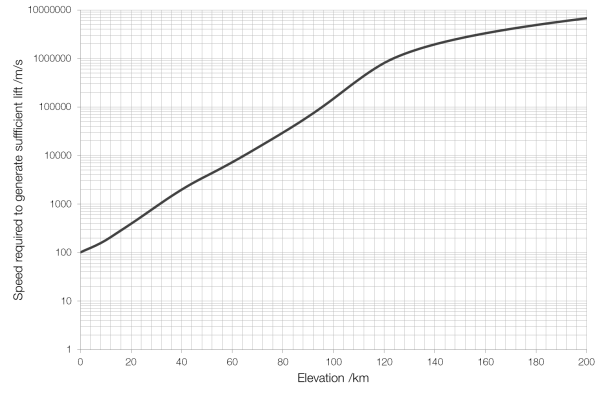Speed required at elevation for a Boeing 747-400.

The cruising speed of a 747-400 is about Mach 0.85 or 290 metres per second, which limits the 747-400 to an elevation of around 16 km. (In reality it flies much lower, around 12?km, for safety and fuel consumption reasons.) The record elevation for sustained horizontal flight by a ground-launched aircraft is 36?240 m by a MiG-25M, which was, at the time, one of the fastest military aircraft with a top speed of Mach 2.83.

The Kármán Line, at an elevation of 100?km, is the point at which the atmosphere is so thin (more than a million times thinner than air at sea level) that an aircraft would have to be moving at a speed faster than it could orbit in order to stay in the air. Thus, it is the greatest altitude at which an aircraft could fly in a straight line rather than following the curvature of the Earth.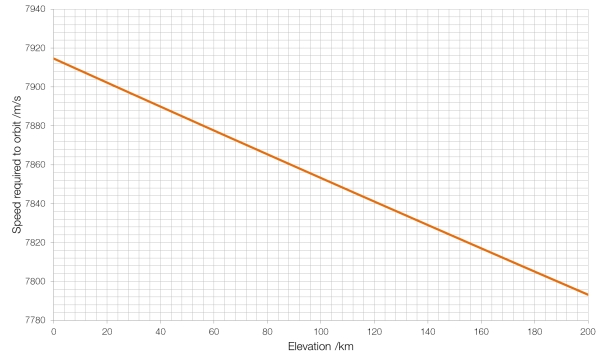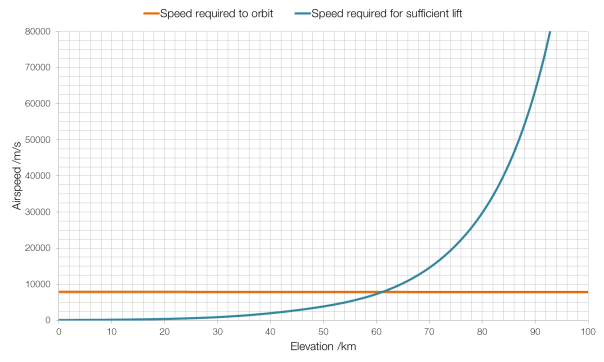As you can see from the graph above, for our Boeing 747-400, the Kármán Line is around 61?km, rather than the full one hundred kilometres, because the 747-400 is not the most aerodynamic of aircraft, designed for bulk passenger carrying and cost-efficiency rather than performance.

# Throats, Nozzles and Shock Diamonds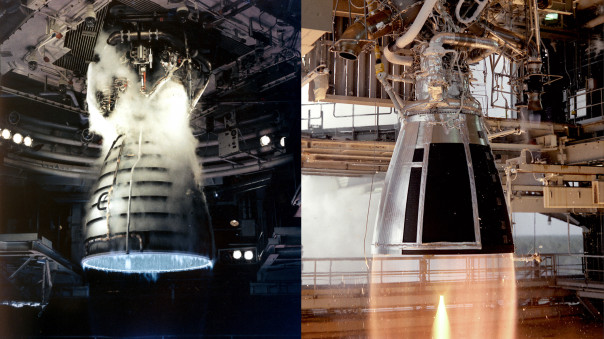All rocket engines feature a “throat”, a narrowing of the exhaust nozzle. In the photographs above this throat is obvious at the top of the images.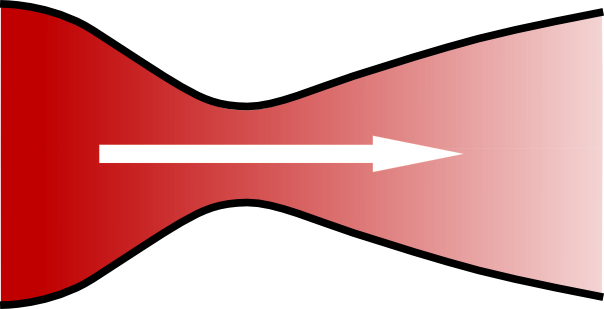A de Laval nozzle (also known as a convergent-divergent nozzle) as used in rocket engines.

The question of why rocket engines all feature throats might seem like it has an obvious answer: just try blowing air out of your mouth with your mouth wide open. But the physics behind rocket throats is a little bit more complicated than that.

The nozzle of a rocket engine is designed to accelerate exhaust gases to Mach 1 at the throat, causing a process known as choking or choked flow.

Normally the rate at which a gas can flow out of a pressurised container, such as the combustion chamber of a rocket engine, is limited by the difference between the pressure of the interior of the container and the pressure of the atmosphere surrounding the container: the container tries to push gas out, and the atmosphere tries to push the gas back in. In choked flow, this dependence disappears: the outside pressure has no effect on the rate at which gas is ejected, external pressure cannot force its way past the supersonic shockwave that forms at the throat. Choked flow produces the greatest rate of flow of exhaust gases, and therefore the highest possible thrust: you cannot get the exhaust gas particles to move any faster, but you can push through more of them per second.

As a gas passes through a narrowing in a pipe its pressure decreases and its speed increases; and as the pipe expands the pressure increases and the speed decreases.* This is known as the Venturi effect.$\frac{dv}{v}=\left( \frac{1}{M^2-1} \right) \frac{dA}{A}$

Where$\frac{dv}{v}$ is the rate of change of the velocity of the gas,$M$ is the speed of the gas as a fraction of the speed of sound (i.e. the gas’s Mach number) and$\frac {dA} {A}$ is the change in the area that the gas is flowing through.

There is a limit to the Venturi effect, and that is when the fluid reaches the (local) speed of sound, as happens during choked flow. At this point, the Venturi effect is reversed: instead of the gas slowing as the nozzle expands, its velocity increases (because the$\frac{1}{M^2-1}$ term becomes positive rather than negative).

Thus the shape of a de Laval nozzle is designed to first accelerate the gas (by narrowing) to sonic speeds at the throat, and then because the Venturi effect has been reversed, to accelerate the gas further (by expanding) to supersonic speeds. The faster the exiting exhaust gas is, the more thrust that will be produced.

Once the gas has exited the throat, the shape of the diverging (widening) part of the de Laval nozzle is such that the exhaust gases are directed backwards parallel to the body of the rocket (as shown in the middle diagram below), giving the maximum possible thrust in the direction that you want the rocket is to travel in.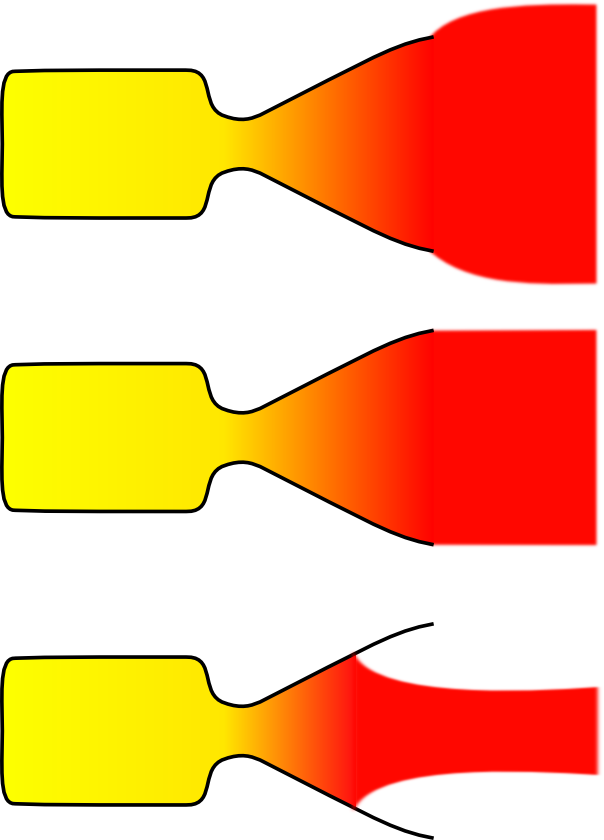An underexpanded nozzle (top) is inefficient because some of the exhaust gas is propelled backwards at an angle to the rocket’s direction of travel, i.e. pushing it right/left and back/forth rather than up. An overexpanded nozzle (bottom) is more efficient than either of the previous two, but the jet of exhaust that it produces is unstable, which could lead to your rocket veering off course.

The degree of expansion of your nozzle depends on the ambient pressure, and so nozzles are often overexpanded at low altitudes and underexpanded at very high altitudes, giving a “sweet spot” during its journey where it operates at the best possible efficiency.

When a nozzle is over- or underexpanded, a complex process can cause shock waves to form in the exhaust flow. Unburnt fuel passing through these shock waves is compressed and burnt, causing bright “shock diamonds” to form.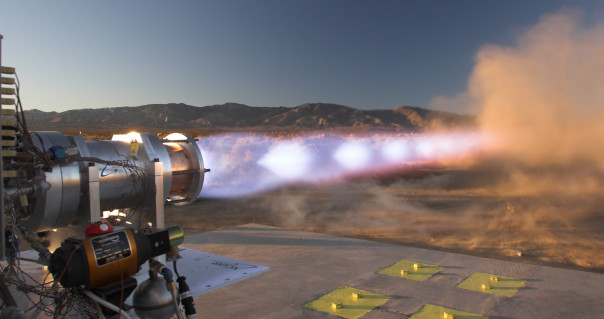Shock diamonds in the exhaust of the XCOR Liquid Oxygen-Methane engine.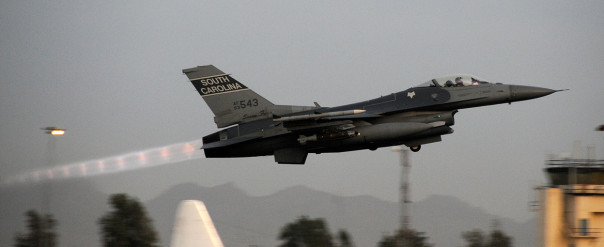Shock diamonds in the exhaust of an F-16 during takeoff.

* This is an interesting example of the conservation of energy: the energy of the fluid is a combination of the fluid’s kinetic and potential energies, and as the speed (and therefore the kinetic energy) of the fluid increases, the potential energy (i.e. its pressure) must decrease.